### Malaysia Math Kangaroo 2014

1. Part A: Each correct answer is worth 3 points

A lady bug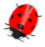will sit on a flower that has five petals and three leaves. On which of the following flowers will the ladybug sit?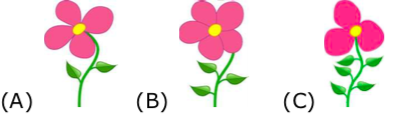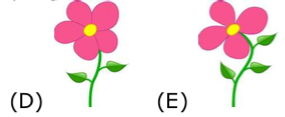2. In what order do you meet the shapes starting from the arrow?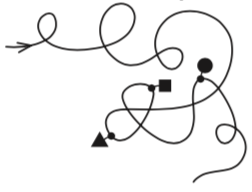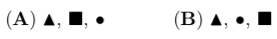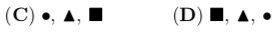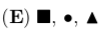3. How many more grey squares than white ones can you see?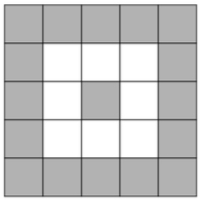4. Put the animials in line from the smallest to the largest. What animal is in the middle?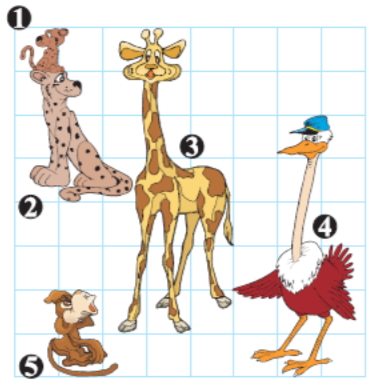5. Ann has twelve of these tiles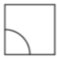. She makes one line with the design. Ann starts at the left side. How does the line end?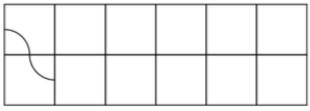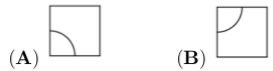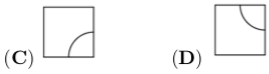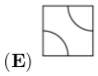6. Which is the shadow of the girl?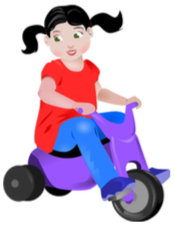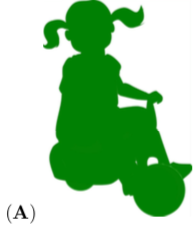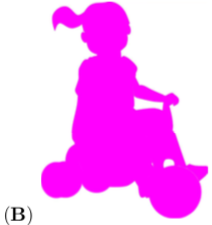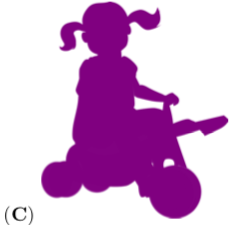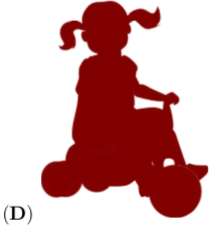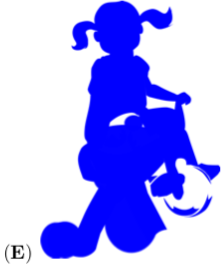7. A square was made of 25 small squares, but some of these small squares were lost. How many small squares were lost?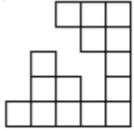8. Part B: Each correct answer is worth 4 points

The first two scales in the picture are balanced. How many ducks are needed on the right side of the third scale, to balance with the crocodile?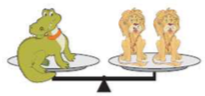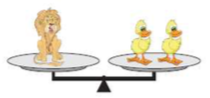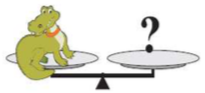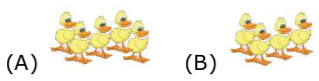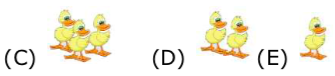9. When the ant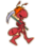goes from home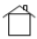following these arrows: → 3, î 3, → 3, î 1, it comes to the ladybug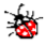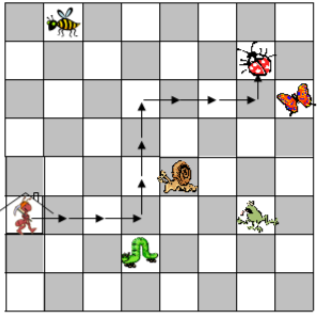Which animal would it come to, if it goes from home following these arrows: → 2, ↓ 2, → 3, ↑ 3, → 2, ↑ 2?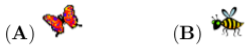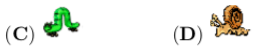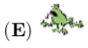10. The kangaroo is inside how many circles?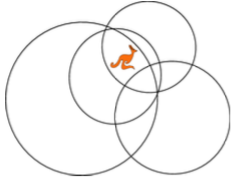11. A square was cut into four parts as shown in the picture.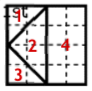Which of the following shapes cannot be made with these 4 parts?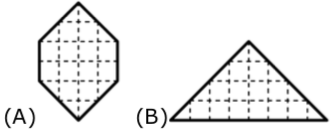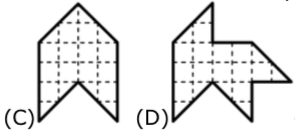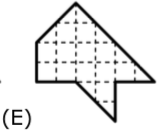12. Which of the shapes below should be placed on top of the shape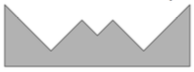to make a rectangle?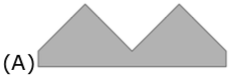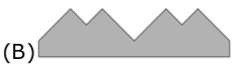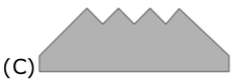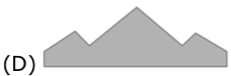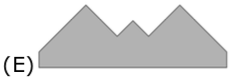13. Walking from K to O along the lines pick up the letters KANGAROO in the correct order. What is the lenght of the shortest walk in meters?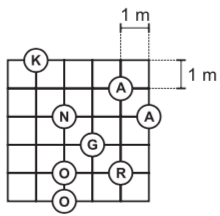14. How many numbers are greater than 10 and less than or equal to 31 which can be written with digits 1, 2 or 3 only? You can repeat digits

15. Seven sticks lie on top of each other. Stick number 2 is at the bottom. Stick number 6 is at the top. Which stick is in the middle?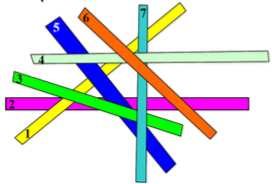16. How many frogs did the three pelicans catch?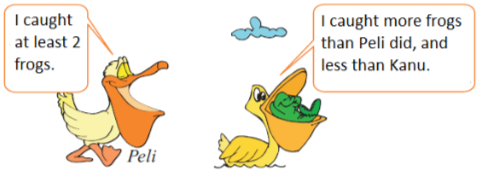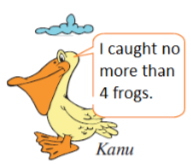17. The chess board is damaged. How many black squares on the right side of the line are missing?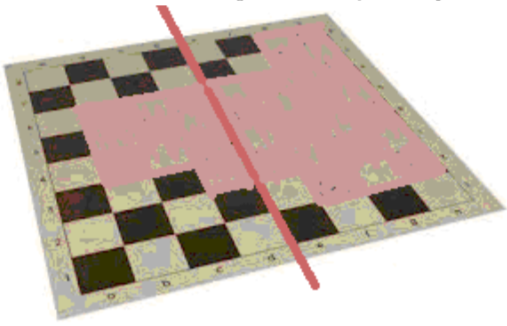18. My rabbit eats only cabbage and carrots. Last week he ate either 10 carrots or 2 heads of cabbage each day. If he ate a total of 6 heads of cabbage last week, how many carrots did he eat?19. What should you put in the square to get a correct diagram?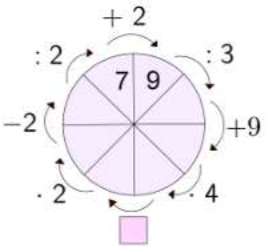20. Place the digits 2, 3, 4, and 5 in the squares so that the sum is as large as possible. What is this sum?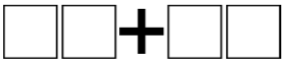21. The central cell of the square was removed. We cut it into equal pieces. Which piece is not possible to get?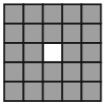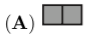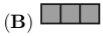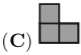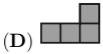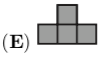22. To get the product of 2 x 3 x 15, Bill has to press the keys of his calculator seven times: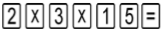.
Bill wants to multiply all numbers from 3 to 21, using his calculator. At least, how many times will he press the keys of his calculator?

23. Fedya has 4 red cubes, 3 blue cubes, 2 green cubes, and 1 yellow cube. He is building a tower (see the picture) in such a way that no two cubes touching each other have the same colour. What is the colour of the cube with the question mark?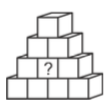24. Gear A is about to make one complete turn in the direction shown in Figure 1.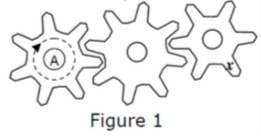The piece “x” moved to one of the positions a, b, c, d, e shown in Figure 2.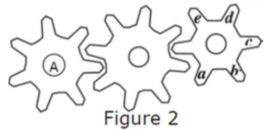To which position did “x” move?